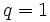# Iwahori-Hecke algebra of symmetric group:S2

(diff) ← Older revision | Latest revision (diff) | Newer revision → (diff)

Note that although the symmetric group$S_2$ is isomorphic as an abstract group to cyclic group:Z2, it is important that we are thinking of it as a symmetric group in this case since the definition of Iwahori-Hecke algebra of the symmetric group relies on thinking of the group as a symmetric group.

The Iwahori-Hecke algebra of symmetric group:S2 over a commutative unital ring$R$ is defined as the$R[q]$-algebra:$R[q]\langle T \rangle/ \langle (T - q)(T + 1) \rangle$

In other words, as a$R[q]$-algebra, it is generated by a single element$T$ which satisfies the relation$(T - q)(T + 1) = 0$, or$T^2 = q + (q - 1)T$.

Specializing$q$ to a particular numerical value$q_0 \in R$ means considering a$R$-algebra obtained by quotienting the base ring by the ideal$\langle q - q_0 \rangle$, i.e., setting$q = q_0$ downstairs.

Specializing to$q = 1$ gives the group algebra over$R$ of the symmetric group, i.e.:$R[S_2] \cong R[T]/(T^2 - 1)$

The multiplication table in general is given as follows:

Identity element,$1$, or$()$$T$ or$(1,2)$$1$ or$()$$1$$T$$T$ or$(1,2)$$T$$q + (q - 1)(T)$

## Interpretation over fields

Specializing to$q$ a prime power gives the Hecke algebra of the general linear group for the general linear group of degree two over the finite field with$q$ elements. The basis$\{ 1, T \}$ represents the set of possible relative positions of pairs of complete flags in a two-dimensional vector space over$\mathbb{F}_q$. Two flags have relative position$1$ if they are identical, and$T$ otherwise. We can now interpret the multiplication as follows: